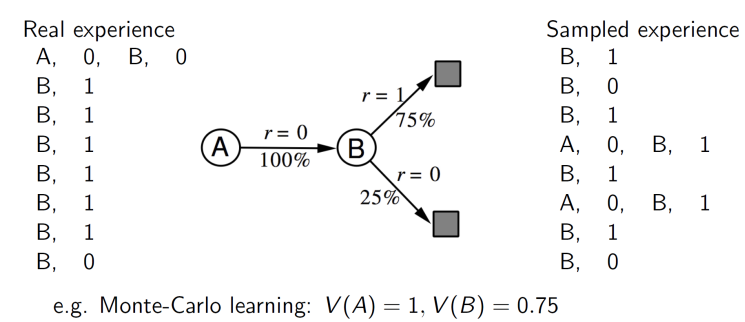# 强化学习概论2

https://morvanzhou.github.io/tutorials/machine-learning/reinforcement-learning/1-1-B-RL-methods/

## Model-free和Model-based

Model-free在真实环境中学习，一步一步根据真实环境的反馈，并根据反馈采取下一步的行动。Model-based在前者的基础上可以对真实环境进行建模从而创建虚拟环境，模型可以在虚拟环境中进行强化。该模型 M \mathcal{M} 通过参数化 η \eta 来表示马尔科夫决策过程MDP &lt; S , A , P , R &gt; \mathcal{&lt;S,A,P,R&gt;}

• S \mathcal{S} :状态空间
• A \mathcal{A} :动作空间
• P \mathcal{P} :状态转移概率 P ( s t + 1 ∣ s t , a t ) P(s_{t+1}|s_{t},a_{t})
• R \mathcal{R} :奖励函数 R ( s t , a t ) R(s_{t},a_{t})

S 1 , A 1 → R 2 , S 2 S 2 , A 2 → R 3 , S 3 . . . . . . S T − 1 , A T − 1 → R T , S T S_{1},A_{1}\rightarrow R_{2},S_{2}\\ S_{2},A_{2}\rightarrow R_{3},S_{3}\\ ......\\ S_{T-1},A_{T-1}\rightarrow R_{T},S_{T}

• 最简单的是查找表模型Table Lookup Model，是一个显式的MDP,根据真实经历组织一个查找表。 由此奖励和概率通过直接求平均和数数量可得到：
P ( s ′ ∣ s , a ) = 1 N ( s , a ) ∑ t = 1 T 1 ( S t , A t , S t + 1 = s , a , s ′ ) R ( s , a ) = 1 N ( s , a ) ∑ t = 1 T 1 ( S t , A t = s , a ) R t P(s&#x27;|s,a)=\frac{1}{N(s,a)}\sum_{t=1}^{T}1(S_{t},A_{t},S_{t+1}=s,a,s&#x27;)\\ R(s,a)=\frac{1}{N(s,a)}\sum_{t=1}^{T}1(S_{t},A_{t}=s,a)R_{t}

Sample-based方法，使用model-free RL来抽样经历，这种方法更有效率。model-based缺点：
model-based RL受限于近似的MDP，所以不能达到最优策略。当model不准确时，最终将是一个局部策略。解决方法：（1）当模型错误，使用model-free RL。（2）明确解释模型的不确定性。

Policy-based-RL与Value-based-RL

Value-based-RL适合离散取值的行动，而连续取值的动作只能采取Policy-based-RL，可以基于概率密度函数取得概率。03-0403-167618
09-042654
09-05810
03-241925
02-13459
10-161670
04-15764
04-01534
03-16776
03-271001
04-153696
02-17137
09-07490
02-13
©️2020 CSDN 皮肤主题: 编程工作室 设计师:CSDN官方博客